### 上海大众 辉昂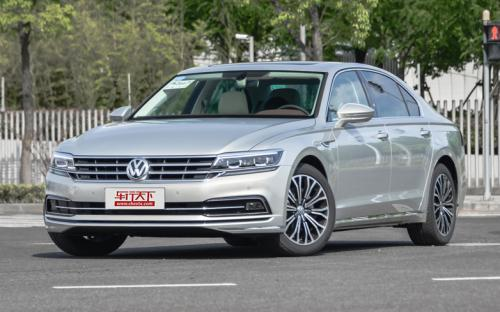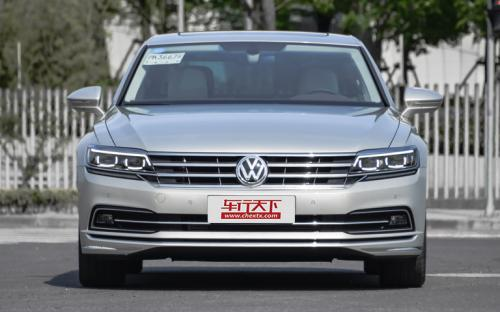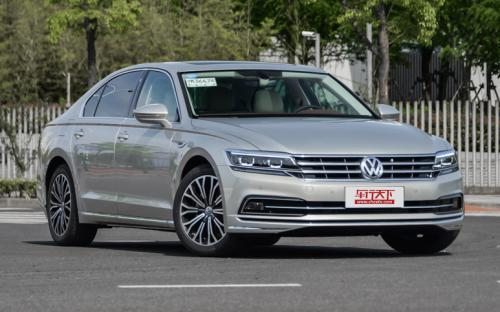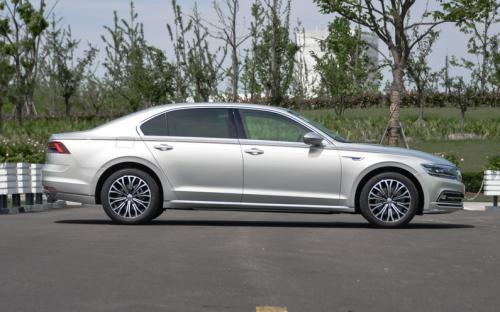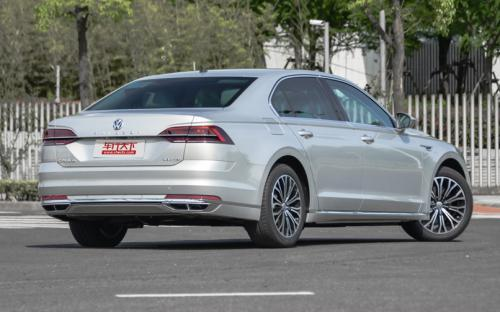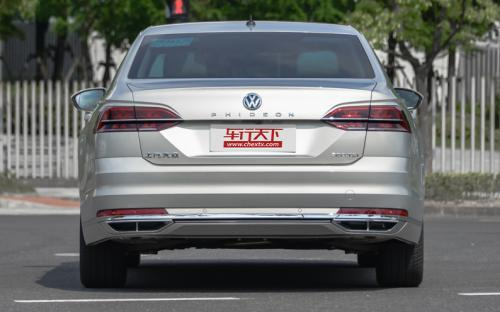0 种颜色可选2016款最低售价：34.90 万元起

5074(mm)1893(mm)1489(mm)##### 配置亮点：
• 胎压监测装置

• ISOFIX儿童座椅接口

• 车身稳定控制(ESC/ESP/DSC等)

• 电动天窗

• 定速巡航

• 后倒车雷达

• 真皮座椅

• GPS导航系统

• 氙气大灯

• 后视镜加热

• 提交
2016款 480 V6 四驱行政旗舰版 (393张)
• 2016款 480 V6 四驱行政旗舰版 (393张)
• 2016款 380TSI 两驱至尊版 (83张)
• 上海大众 辉昂 绕车实拍• 上海大众 辉昂 在售车型

排量 车型 厂商指导价 本地最低报价 购车工具
2.0T
380TSI 两驱御尊版 7挡双离合
34.90万
34.90万

38.90万
380TSI 两驱至尊版 7挡双离合
40.90万
380TSI 两驱行政版 7挡双离合
46.90万
46.90万

53.90万
480 V6 四驱至尊版 7挡双离合
52.90万
480 V6 四驱行政版 7挡双离合
58.90万
65.90万
65.90万

3.0T
380TSI 两驱御尊版 7挡双离合
34.90万
34.90万

38.90万
380TSI 两驱至尊版 7挡双离合
40.90万
380TSI 两驱行政版 7挡双离合
46.90万
46.90万

53.90万
480 V6 四驱至尊版 7挡双离合
52.90万
480 V6 四驱行政版 7挡双离合
58.90万
65.90万
65.90万

上海大众 辉昂 经销商

查看更多 >>

### 上海大众 辉昂 动力加速

辉昂 0-100公里加速时间分布在 6.3-8.1秒 属于 高性能级

动力级别 加速时间 车型
运动级(1款)
8.1s
380TSI 两驱御尊版 380TSI 两驱御尊豪华版380TSI 两驱至尊版380TSI 两驱行政版380TSI 两驱行政旗舰版
高性能级(1款)
6.3s
480 V6 四驱至尊版480 V6 四驱行政版480 V6 四驱行政旗舰版

上海大众 辉昂 视频

上海大众 辉昂 新闻资讯

# 上汽大众辉昂正式上市 售价34.90万元起

国产新车 超过9725次关注

2016年10月21日，备受瞩目的上汽大众辉昂PHIDEON正式上市发售。据悉，定位于中大型豪华轿车的辉昂在分别搭载2.0T涡轮增压发动机及3.0TV6机械增压发动机基础上根据配...

# 旗舰升级 上汽大众辉昂或于十月上市

国产新车 超过9608次关注

日前，我们从官方获悉，上汽大众全新中大型车辉昂将于今年10月份正式上市。新车已在北京车展前夕的大众之夜上亮相，动力上将搭载3.0T或者2.0T发动机，提供前驱或者...

猜你喜欢

﻿
• 快速找车
• 选择品牌
• 选择品牌
• A  奥迪
• A  阿斯顿·马丁
• A  阿尔法·罗密欧
• B  宝沃
• B  布加迪
• B  巴博斯
• B  保时捷
• B  宾利
• B  奔驰
• B  宝马
• B  本田
• B  别克
• B  标致
• B  比亚迪
• B  宝骏
• B  北汽制造
• B  北汽新能源
• B  北汽幻速
• B  北汽威旺
• B  北京汽车
• B  奔腾
• B  北汽绅宝
• C  长安
• C  长安商用
• C  长城
• C  昌河
• D  大众
• D  道奇
• D  DS
• D  东南
• D  东风风神
• D  东风风行
• D  东风小康
• D  东风风度
• D  东风
• F  福特
• F  丰田
• F  菲亚特
• F  法拉利
• F  福田
• F  福迪
• F  福汽启腾
• G  观致
• G  广汽传祺
• G  广汽吉奥
• G  GMC
• H  红旗
• H  汉腾汽车
• H  哈弗
• H  哈飞
• H  海格
• H  海马
• H  华颂
• H  黄海
• H  华泰
• H  恒天
• J  吉利汽车
• J  捷豹
• J  Jeep
• J  江淮
• J  江铃
• J  金杯
• J  九龙
• J  金旅
• K  凯翼
• K  凯迪拉克
• K  克莱斯勒
• K  科尼塞克
• K  卡威
• K  开瑞
• L  路虎
• L  林肯
• L  劳斯莱斯
• L  兰博基尼
• L  雷克萨斯
• L  铃木
• L  雷诺
• L  理念
• L  力帆
• L  莲花汽车
• L  猎豹
• L  路特斯
• L  陆风
• M  马自达
• M  MG
• M  MINI
• M  玛莎拉蒂
• M  摩根
• M  迈凯轮
• N  纳智捷
• O  欧宝
• O  讴歌
• O  欧朗
• Q  奇瑞
• Q  起亚
• Q  启辰
• R  日产
• R  荣威
• R  瑞麒
• S  三菱
• S  斯威汽车
• S  萨博
• S  smart
• S  斯柯达
• S  斯巴鲁
• S  思铭
• S  双龙
• S  上汽大通
• S  双环
• T  特斯拉
• T  腾势
• W  沃尔沃
• W  五菱汽车
• W  五十铃
• W  威兹曼
• W  威麟
• X  现代
• X  雪佛兰
• X  雪铁龙
• X  西雅特
• Y  一汽
• Y  英菲尼迪
• Y  英致
• Y  依维柯
• Y  野马汽车
• Y  永源
• Z  众泰
• Z  中华
• Z  中兴
• Z  知豆
• 选择车系
• 选择车系
• 车型对比
• 选择品牌
• 选择品牌
• A  奥迪
• A  阿斯顿·马丁
• A  阿尔法·罗密欧
• B  宝沃
• B  布加迪
• B  巴博斯
• B  保时捷
• B  宾利
• B  奔驰
• B  宝马
• B  本田
• B  别克
• B  标致
• B  比亚迪
• B  宝骏
• B  北汽制造
• B  北汽新能源
• B  北汽幻速
• B  北汽威旺
• B  北京汽车
• B  奔腾
• B  北汽绅宝
• C  长安
• C  长安商用
• C  长城
• C  昌河
• D  大众
• D  道奇
• D  DS
• D  东南
• D  东风风神
• D  东风风行
• D  东风小康
• D  东风风度
• D  东风
• F  福特
• F  丰田
• F  菲亚特
• F  法拉利
• F  福田
• F  福迪
• F  福汽启腾
• G  观致
• G  广汽传祺
• G  广汽吉奥
• G  GMC
• H  红旗
• H  汉腾汽车
• H  哈弗
• H  哈飞
• H  海格
• H  海马
• H  华颂
• H  黄海
• H  华泰
• H  恒天
• J  吉利汽车
• J  捷豹
• J  Jeep
• J  江淮
• J  江铃
• J  金杯
• J  九龙
• J  金旅
• K  凯翼
• K  凯迪拉克
• K  克莱斯勒
• K  科尼塞克
• K  卡威
• K  开瑞
• L  路虎
• L  林肯
• L  劳斯莱斯
• L  兰博基尼
• L  雷克萨斯
• L  铃木
• L  雷诺
• L  理念
• L  力帆
• L  莲花汽车
• L  猎豹
• L  路特斯
• L  陆风
• M  马自达
• M  MG
• M  MINI
• M  玛莎拉蒂
• M  摩根
• M  迈凯轮
• N  纳智捷
• O  欧宝
• O  讴歌
• O  欧朗
• Q  奇瑞
• Q  起亚
• Q  启辰
• R  日产
• R  荣威
• R  瑞麒
• S  三菱
• S  斯威汽车
• S  萨博
• S  smart
• S  斯柯达
• S  斯巴鲁
• S  思铭
• S  双龙
• S  上汽大通
• S  双环
• T  特斯拉
• T  腾势
• W  沃尔沃
• W  五菱汽车
• W  五十铃
• W  威兹曼
• W  威麟
• X  现代
• X  雪佛兰
• X  雪铁龙
• X  西雅特
• Y  一汽
• Y  英菲尼迪
• Y  英致
• Y  依维柯
• Y  野马汽车
• Y  永源
• Z  众泰
• Z  中华
• Z  中兴
• Z  知豆
• 选择车系
• 选择车系
• 选择车型
• 选择车型
• 意见反馈